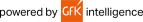Shopping Cart:
0 Item
Total:

View Cart/Checkout

Scientific Calculator, Twin Power, 3-1/8"x6"x2/5"

469-function dual power scientific calculator is ideal for students studying general math, algebra, geometry, and trigonometry. Features two-line display with nine memories and last answer recall two variable statistics, binary/octal/hexadecimal calculations, differential and integral calculus, 44 metric and 52 scientific conversions, fraction calculations. Multi-line playback feature makes scientific equations easier for students to solve. After getting an answer, the student may then "playback" the expressions and substitute new numeric values. Direct algebraic logic allows students to enter the elements of an expression in the exact order they appear in the textbook. Twin power scientific calculator automatically switches from solar power to battery power in low light. Hard cover protects calculator.

41014-00  |  Mfr: EL546XBWH  |
General Information
Product Type : Scientific Calculator
Brand Name : Sharp Calculators
Manufacturer : Sharp Calculators
Product Model : EL-546XBWH
Product Name : EL546X Scientific Calculator
Manufacturer Part Number : EL546XBWH

Physical Characteristics
Height : 0.4"
Width : 3.1"

Product Information
Length : 6"

Display & Graphics
Number of Display Lines : 2
Number of Display Digits : 10

Battery Information
Power Source : Battery/Solar

Technical Information
Operations/Functionality :
• Memory
• Recall
• Variable Statics
• Binary
• Octal
• Differential Calculus
• Integral Calculus
• Metric Conversion
• Scientific Conversion
• Fraction Conversion
• Payback
• Scientific Equation
• Substitution
• Algebra
• Auto Power Off

Number of Functions : 469Consider:EACH

41014-00 -
\$49.99 Net